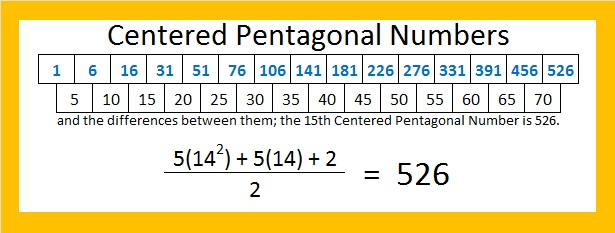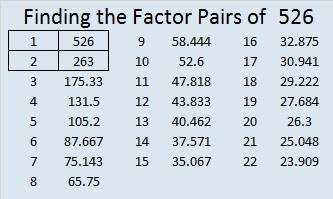# 526 is a Centered Pentagonal Number

I am excited that Kerrydunton included the Find the Factors puzzles on a list of great maths starters.

1 + 5 + 10 + 15 + 20 + 25 + 30 + 35 + 40 + 45 + 50 + 55 + 60 + 65 + 70 = 526. Thus, 526 is the 15th centered pentagonal number.

The above equation is the same as saying that 526 is one more than five times the 14th triangular number.The last digit of a centered pentagonal number is always 1 or 6. Triangular numbers greater than 3 are always composite numbers, but centered pentagonal numbers ending in 1 might be prime numbers. For example 31, 181, 331, 601, 1051, 1381, and 3331 are centered pentagonal numbers and prime numbers.Print the puzzles or type the solution on this excel file: 12 Factors 2015-06-15

—————————————————————————————————

• 526 is a composite number.
• Prime factorization: 526 = 2 x 263
• The exponents in the prime factorization are 1 and 1. Adding one to each and multiplying we get (1 + 1)(1 + 1) = 2 x 2 = 4. Therefore 526 has exactly 4 factors.
• Factors of 526: 1, 2, 263, 526
• Factor pairs: 526 = 1 x 526 or 2 x 263
• 526 has no square factors that allow its square root to be simplified. √526 ≈ 22.93468988—————————————————————————————————## 3 thoughts on “526 is a Centered Pentagonal Number”

1.Steve Morris

It’s intriguing how so many numbers have interesting or special properties. I used to think that there was something magical about this, that these patterns were somehow telling us something deep about the universe. Like, why is 3.14159… the value it is?

Nowadays I think that these special properties are things we invented. For instance, a centred pentagonal number is interesting if you think it is, and isn’t if you don’t think so. Some numbers have particularly important or curious properties (prime numbers for instance), but they are only interesting because we think they are!

What do you think?

•ivasallay

This is an intriguing comment. My response was a little longer than usual so I decided to write a post instead. I’ll publish it in a couple of days. Thanks for getting me thinking!

•Steve Morris

I’m looking forward to that!!

This site uses Akismet to reduce spam. Learn how your comment data is processed.